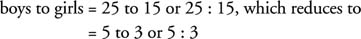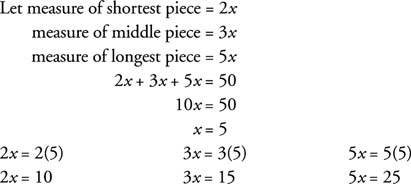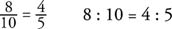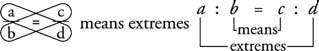## Ratio and Proportion

Ratio is a concept that you have probably encountered in other math classes. It is a comparison of sizes.

## Ratio

The ratio of two numbers a and b is the fraction, usually expressed in reduced form. An alternative form involves a colon. The colon form is most frequently used when comparing three or more numbers to each other. See Table .Example 1: A classroom has 25 boys and 15 girls. What is the ratio of boys to girls?The ratio of boys to girls is 5 to 3, or 5/3, or 5 : 3.

Example 2: The ratio of two supplementary angles is 2 to 3. Find the measure of each angle.

• The angles have measures of 72° and 108°.
• 2x to 3x reduces to 2 to 3.
• 2x + 3x = 180° (The sum of supplementary angles is 180°.)
• x = 180°
• x = 36°
• Then, 2x = 2(36°) and 3x = 3(36°).
• So, 2x = 72° and 3x = 108°
• The angles have measures of 72° and 108°.

Example 3: A triangle has angle measures of 40°, 50°, and 90°. In simplest form, what is the ratio of these angles to each other?

• 40 : 50 : 90 = 4 : 5 : 9 (10 is a common divisor)

This means that:

1. The ratio of the first to the second is 4 to 5.

2. The ratio of the first to the third is 4 to 9.

3. The ratio of the second to the third is 5 to 9.

Example 4: A 50‐inch segment is divided into three parts whose lengths have the ratio 2 : 3 : 5. What is the length of the longest part?The longest part has a measure of 25 inches.

## Proportion

proportion is an equation stating that two ratios are equal.## Means and extremes

The extremes are the terms in a proportion that are the farthest apart when the proportion is written in colon form ( a:b = c:d). In the foregoing, a and d are extremes. The means are the two terms closest to each other.In the preceding proportion, the values a and d are called extremes of the proportion; the values b and c are called the means of the proportion.